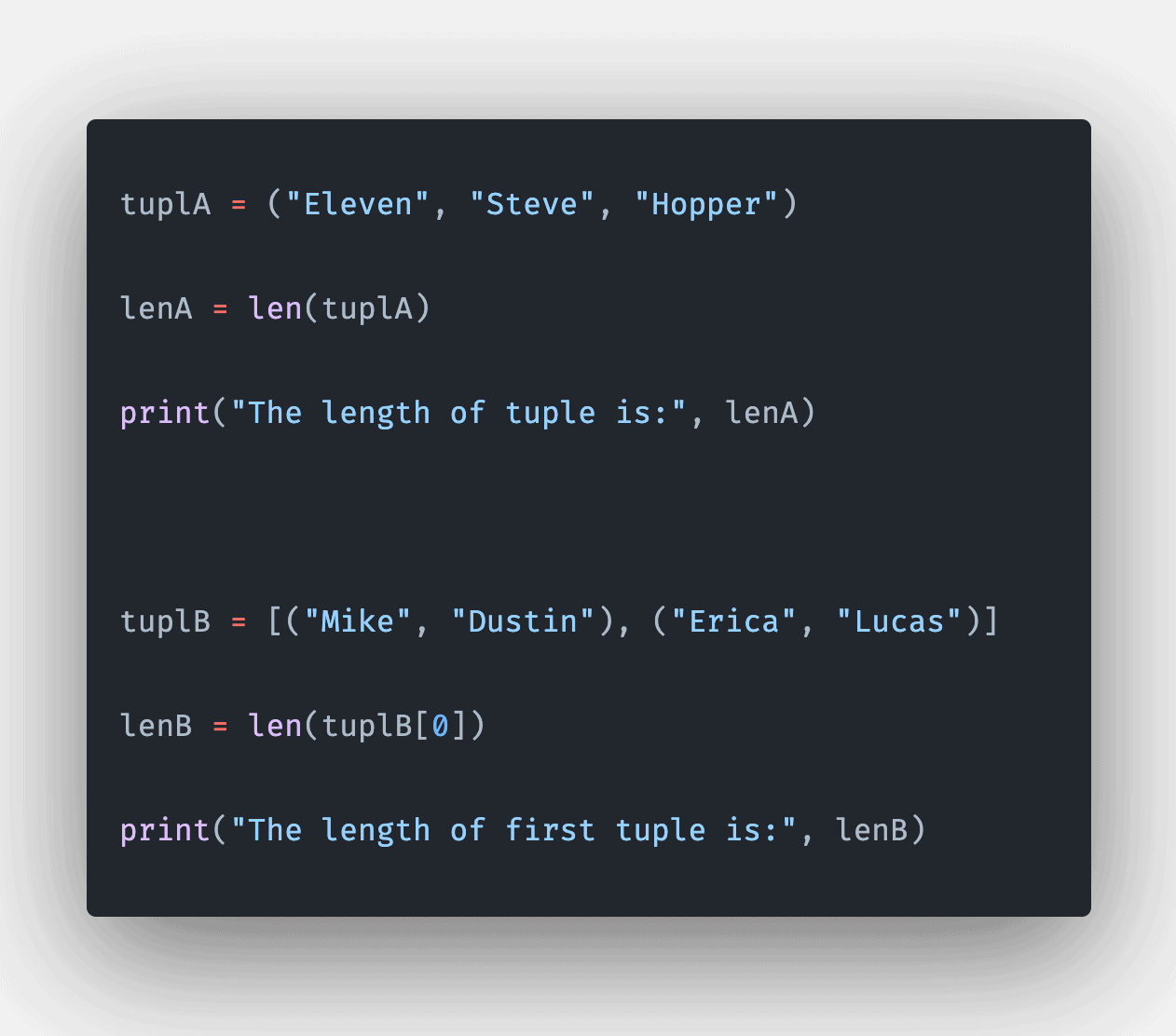# Python tuple length: How to Get Length of Tuple in Python

0
10The tuple is a Python object that has a sequence of values stored that can be of any type, and they are indexed by integers.

## How to Get length of Tuple in Python

To get a length of a tuple in Python, use the len() function. The len() is a built-in function that returns the length (the number of elements) of an object and can be passed as a sequence (a tuple, string, list, range, or bytes) or a collection (a dictionary, set, or frozen set).

``````tuplA = ("Eleven", "Steve", "Hopper")
lenA = len(tuplA)
print("The length of tuple is:", lenA)

tuplB = [("Mike", "Dustin"), ("Erica", "Lucas")]
lenB = len(tuplB)
print("The length of first tuple is:", lenB)``````

#### Output

``````The length of tuple is: 3
The length of first tuple is: 2``````

In the first example, we defined a simple tuple with three elements; hence, it returns 3 as output when we apply the len() function to a tuple.

In the second example, we defined a list of two tuples, and we want to find the length of the first tuple; hence we use the len() function and pass the tuplB.

Python tuple’s index starts with 0. That’s why the index of the first element in the tuple is 0, and the index of the last item is len(tuplA) – 1.

## How to check if a tuple is empty in Python

To check if a tuple is empty in Python, use the len() function with the double equals operator(==).

``````tup = ()

if len(tup) == 0:
print("tuple is empty")
else:
print("tuple is not empty")``````

#### Output

``tuple is empty``

In this example, we are comparing the empty tuple with 0, and if it is, then it means the tuple is empty; otherwise, not. If a tuple has a length of 0, then it’s empty.

## Checking if a tuple is empty using not operator

The not operator in Python allows you to invert the truth value of Boolean expressions and objects. For example, to check if a tuple is empty, negate the condition with not in the if statement.

``````tup = ()

if not tup:
print("tuple is empty")
else:
print("tuple is NOT empty")``````

#### Output

``tuple is empty``

## Conclusion

To get the size of a tuple in Python, use the len() function.

That’s it for this tutorial.

## Related posts

Python list length

Python array length

Python string length

This site uses Akismet to reduce spam. Learn how your comment data is processed.